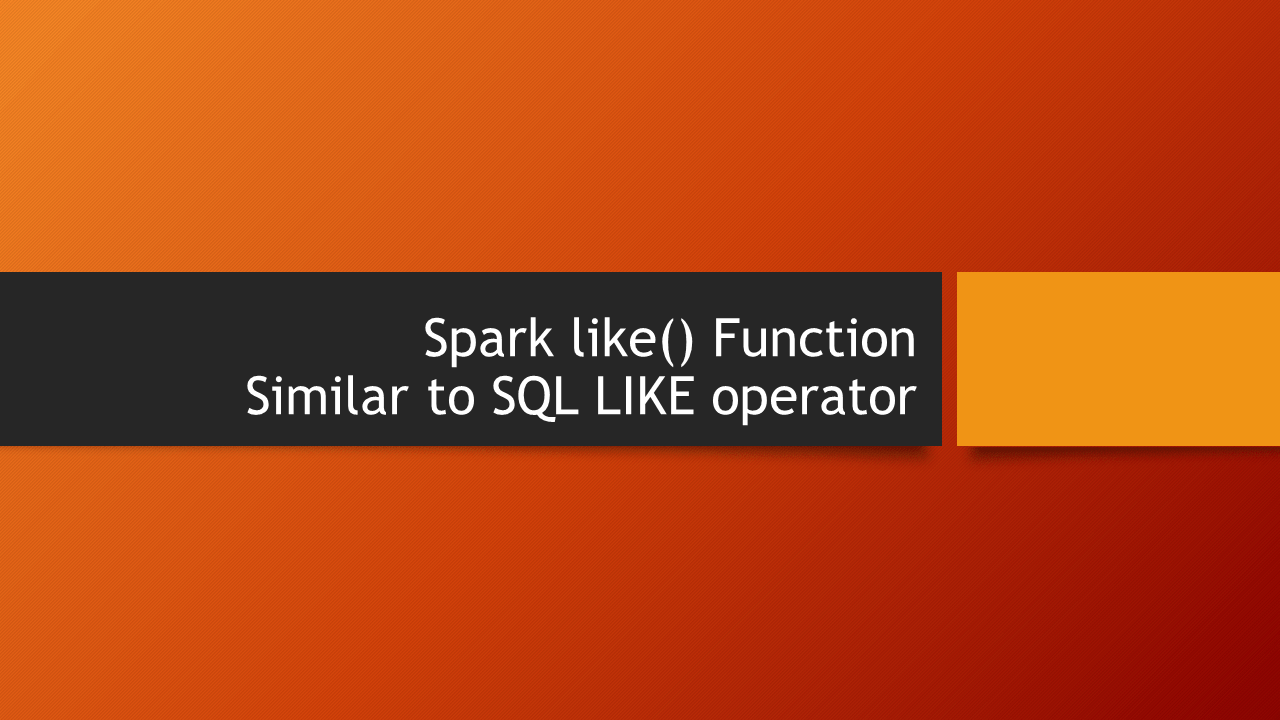# Spark SQL like() Using Wildcard Example

In Spark & PySpark like() function is similar to `SQL LIKE operator` that is used to match based on wildcard characters (percentage, underscore) to filter the rows. You can use this function to filter the DataFrame rows by single or multiple conditions, to derive a new column, use it on when().otherwise() expression e.t.c.

PySpark Example: How to use like() function in PySpark

Below is a quick snippet of using `like()` function on Spark filter, for more examples, refer to below sections. If you wanted to filter by case insensitive refer to filter by regular expression in Spark(Scala) & PySpark(Python).

``````
import org.apache.spark.sql.functions.col
df.filter(col("name").like("%rose%")).show()
``````

`like()` function returns a boolean Column, for more column functions refer to Most used PySpark Column Type Functions.

Spark Column’s like() function accepts only two special characters that are the same as SQL LIKE operator.

• `_` (underscore) – which matches an arbitrary character (single). Equivalent to `?` on shell/cmd
• `%` (percent) – which matches an arbitrary sequence of characters (multiple). Equivalent to `*` on shell/cmd.

## 1. Spark DataFrame like() Function To Filter Rows

Following are few examples of how to use like() function to filter Spark DataFrame rows by using wildcard characters. You can use && and || operators to have multiple conditions in Scala.

Example 1

``````
val data = Seq((1,"James Smith"), (2,"Michael Rose"),
(3,"Robert Williams"), (4,"Rames Rose"),(5,"Rames rose"))
import spark.implicits._
val df = data.toDF("id","name")

// Filter rows that contains 'rose' in 'name' column
import org.apache.spark.sql.functions.col
df.filter(col("name").like("%rose%")).show()

//+---+----------+
//| id|      name|
//+---+----------+
//|  5|Rames rose|
//+---+----------+
``````

Example 2

``````

//Filter rows that starts with R following by any characters
df.filter(col("name").like("R%")).show()

//+---+---------------+
//| id|           name|
//+---+---------------+
//|  3|Robert Williams|
//|  4|     Rames Rose|
//|  5|     Rames rose|
//+---+---------------+
``````

Example 3

``````
// Filter column having values 'Rames _ose',
// Matches any character for _.
df.filter(col("name").like("Rames _ose")).show()

//+---+----------+
//| id|      name|
//+---+----------+
//|  4|Rames Rose|
//|  5|Rames rose|
//+---+----------+
``````

## 2. Spark SQL Using LIKE Operator similar to SQL

Like ANSI SQL, in Spark also you can use LIKE Operator by creating a SQL view on DataFrame, below example filter table rows where `name` column contains `rose` string.

Create Spark temporary view by using `createOrReplaceTempView()`

``````
//Using it on SQL to filter rows
df.createOrReplaceTempView("TAB")
spark.sql("select * from TAB where name like '%rose%'").show()
``````

Refer to above section for more examples.

## 3. PySpark Like() Function Examples

Below is a complete example of using the PySpark SQL like() function on DataFrame columns, you can use the SQL LIKE operator in the PySpark SQL expression, to filter the rows e.t.c

``````
from pyspark.sql import SparkSession
spark = SparkSession.builder.appName('SparkByExamples.com').getOrCreate()
data = [(1,"James Smith"), (2,"Michael Rose"),
(3,"Robert Williams"), (4,"Rames Rose"),(5,"Rames rose")]
df = spark.createDataFrame(data=data,schema=["id","name"])

#Filter where name contains rose string
from pyspark.sql.functions import col
df.filter(col("name").like("%rose%")).show()
``````

Similarly, you can also try other examples explained in above sections.

## Conclusion

In conclusion, Spark & PySpark support SQL LIKE operator by using like() function of a Column class, this function is used to match a string value with single or multiple character by using _ and % respectively.

Happy Learning !!

### Naveen (NNK)

Naveen (NNK) is a Data Engineer with 20+ years of experience in transforming data into actionable insights. Over the years, He has honed his expertise in designing, implementing, and maintaining data pipelines with frameworks like Apache Spark, PySpark, Pandas, R, Hive and Machine Learning. Naveen journey in the field of data engineering has been a continuous learning, innovation, and a strong commitment to data integrity. In this blog, he shares his experiences with the data as he come across. Follow Naveen @ @ LinkedIn